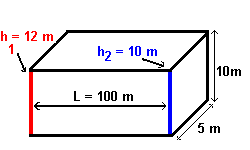## Calculator 1 - Darcy Flux and Average Linear Groundwater Velocity

New Page 1 New Page 1

###Darcy's law is the basic equation that describes fluid flow through porous media.  There are many ways to write Darcy's Law, and a few of them will be presented here.  The first equation contains a velocity term, v (L/T), a conductivity term, K (L/T), a head term, h (L), and a distance term, l (L):

v = -K(∆h/∆l)

This is the Darcy velocity (or Darcy flux) which is defined as the flow per unit cross sectional area of the porous medium. Since you have a porous media the water must move through the pores, around the solid particles, at a speed greater than the flux.  This speed is called the average linear groundwater velocity and is calculated by dividing the Darcy flux by the porosity of the media.DARCY FLUX AND AVERAGE LINEAR GROUNDWATER VELOCITY CALCULATOR

Hydraulic Conductivity:
Effective Porosity (%):

Darcy Flux:
Average Linear Groundwater Velocity

Note: Units of Darcy flux and average linear velocity are the same as those used for conductivityExample:Not to scale

L =100m                     K = 1 x 10-5 m/s       Porosity = 30% or 0.3

A = 5 x 10 = 50m2     dh = (h2 - h1) = (10 - 12) = -2 m

therefore,

i = (-2)/100 = -0.02

v = - (10-5) x (-0.02) = 2 x 10-7 m/s

Q = - (10-5) x (-0.02) x 50 = 1 x 10-5 m3/s

Note: To Calculate Average Linear Velocity which is what we use for groundwater calculations you must divide v by porosity.

Average Linear Velocity

v/porosity = 2 x 10-7 m/s / 0.3  =  6.6 x  10-7   m/s